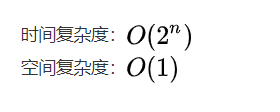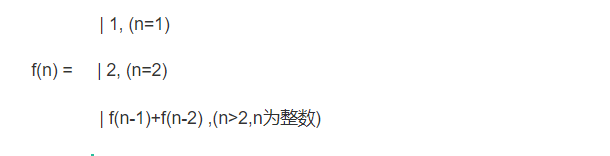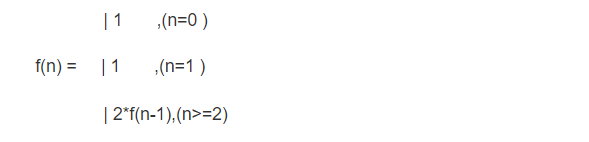# 大家都知道斐波那契数列，现在要求输入一个整数n，请你输出斐波那契数列的第n项（从0开始，第0项为0，第1项是1）。n<=39

public class Solution {
public int Fibonacci(int n) {
if(n<=1){
return n;
}
return Fibonacci(n-1) + Fibonacci(n-2);
}
}# 一只青蛙一次可以跳上1级台阶，也可以跳上2级。求该青蛙跳上一个n级的台阶总共有多少种跳法（先后次序不同算不同的结果）。

a.如果两种跳法，1阶或者2阶，那么假定第一次跳的是一阶，那么剩下的是n-1个台阶，跳法是f(n-1);

b.假定第一次跳的是2阶，那么剩下的是n-2个台阶，跳法是f(n-2)

c.由a\b假设可以得出总跳法为: f(n) = f(n-1) + f(n-2)

d.然后通过实际的情况可以得出：只有一阶的时候 f(1) = 1 ,只有两阶的时候可以有 f(2) = 2

e.可以发现最终得出的是一个斐波那契数列：public class Solution {
public int JumpFloor(int target) {
if (target <= 1){
return 1;
}else {
return JumpFloor(target-1)+JumpFloor(target-2);
}
}
}


# 一只青蛙一次可以跳上1级台阶，也可以跳上2级……它也可以跳上n级。求该青蛙跳上一个n级的台阶总共有多少种跳法。

f(1) = 1

f(2) = f(2-1) + f(2-2) //f(2-2) 表示2阶一次跳2阶的次数。

f(3) = f(3-1) + f(3-2) + f(3-3)

f(n) = f(n-1) + f(n-2) + f(n-3) + … + f(n-(n-1)) + f(n-n)

1）这里的f(n) 代表的是n个台阶有一次1,2,…n阶的 跳法数。

2）n = 1时，只有1种跳法，f(1) = 1

1. n = 2时，会有两个跳得方式，一次1阶或者2阶，这回归到了问题（1） ，f(2) = f(2-1) + f(2-2)

2. n = 3时，会有三种跳得方式，1阶、2阶、3阶，

那么就是第一次跳出1阶后面剩下：f(3-1);第一次跳出2阶，剩下f(3-2)；第一次3阶，那么剩下f(3-3)

因此结论是f(3) = f(3-1)+f(3-2)+f(3-3)

3. n = n时，会有n中跳的方式，1阶、2阶…n阶，得出结论：

f(n) = f(n-1)+f(n-2)+…+f(n-(n-1)) + f(n-n) => f(0) + f(1) + f(2) + f(3) + … + f(n-1)

4. 由以上已经是一种结论，但是为了简单，我们可以继续简化：

f(n-1) = f(0) + f(1)+f(2)+f(3) + … + f((n-1)-1) = f(0) + f(1) + f(2) + f(3) + … + f(n-2)

f(n) = f(0) + f(1) + f(2) + f(3) + … + f(n-2) + f(n-1) = f(n-1) + f(n-1)

可以得出：

f(n) = 2*f(n-1)

5. 得出最终结论,在n阶台阶，一次有1、2、…n阶的跳的方式时，总得跳法为：public class Solution {
public int JumpFloorII(int target) {
if (target <= 0) {
return -1;
} else if (target == 1) {
return 1;
} else {
return 2 * JumpFloorII(target - 1);
}
}
}

09-103655
08-29141206-022837
08-164万+
11-025万+
12-19
08-051万+
10-241万+
08-061万+